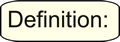Accuracy in Measurement MathBitsNotebook.com Terms of Use   Contact Person: Donna RobertsAccuracy is a measure of how close the result of a measurement comes to the true, actual, or accepted measurement of the object. How close is your measurement to the known measurement of the object?

Any measurement made with a measuring device is approximate.
If you measure the same object two different times, the two measurements may not be exactly the same.

Some limitations in measurements are the result of the measuring instruments.
Such limitations of the instrument can produce results that differ from the true values.
The more precise the measuring instrument, the more accurate the reading.

Example: Accurate Measurements: You purchase a notepad where the packaging states that the pad is 8.5 inches long. You measure the pad three times and get measurements of 8.4 in., 8.6 in., and 8.7 in. Your measurements are very close to the actual measurement and would be considered to be quite accurate. The closer your measurements are to the actual measurement stated on the package, the more accurate your measurements will be.

Example: Measuring Limitations: The width of a quarter measures 24.3 millimeters. When you measure the quarter you use a ruler that contains only centimeter markings. You measure the quarter to be 2.5 centimeters, which is a close approximation and quite an accurate measurement, but it is not the true width of the quarter. You are limited in finding the exact width by the use of an instrument incapable of showing more accurate readings.Accuracy Rules for mathematical operations: When carrying out calculations, the general rule is that the accuracy of a calculated result is limited by the least accurate measurement involved in the calculation.

The greatest degree of accuracy for a result is based on
the least precise measurement used.

Example:
If the units in the calculation are in tenths and hundredths, then the level of accuracy will be in tenths.
18.5 inches added to 24.22 inches = 42.72 inches. Accuracy: 42.7 inches.
A measurement in tenths is less accurate than a measurement in hundredths,
so our solution will only be as good as the least accurate measurement used.

You cannot improve the accuracy by doing arithmetic with measurements.

Calculation should never be carried out to a greater accuracy
than that shown in the original measurements.

Example: If you multiply \$24.56 by 35% off, you will get a savings of 24.36 x 0.35 = 8.526.
We know that money is always rounded to the nearest penny = \$8.53.
Both values in the problem are expressed in hundredths,
and the answer is expressed in hundredths.